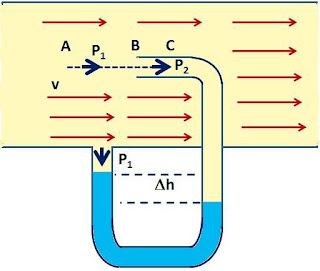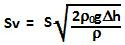## Thursday, May 7, 2009

### Irodov Problem 1.317The streamlines of the gas are depicted by the straight line arrows indicated in the figure. Consider the a line in the path of the gas ABC. At points between A through B along this line the velocity of the gas is v. However, along point between B to C, the velocity of the gas is 0, since it has nowhere to flow.

Bernoulli's equation must apply to all points along a line ABC. Let the pressure of the gas in the region AB be P1 and that in the region BC (inside the pilot tube) be P2.

Hence, we have,The total amount of flow is given by,